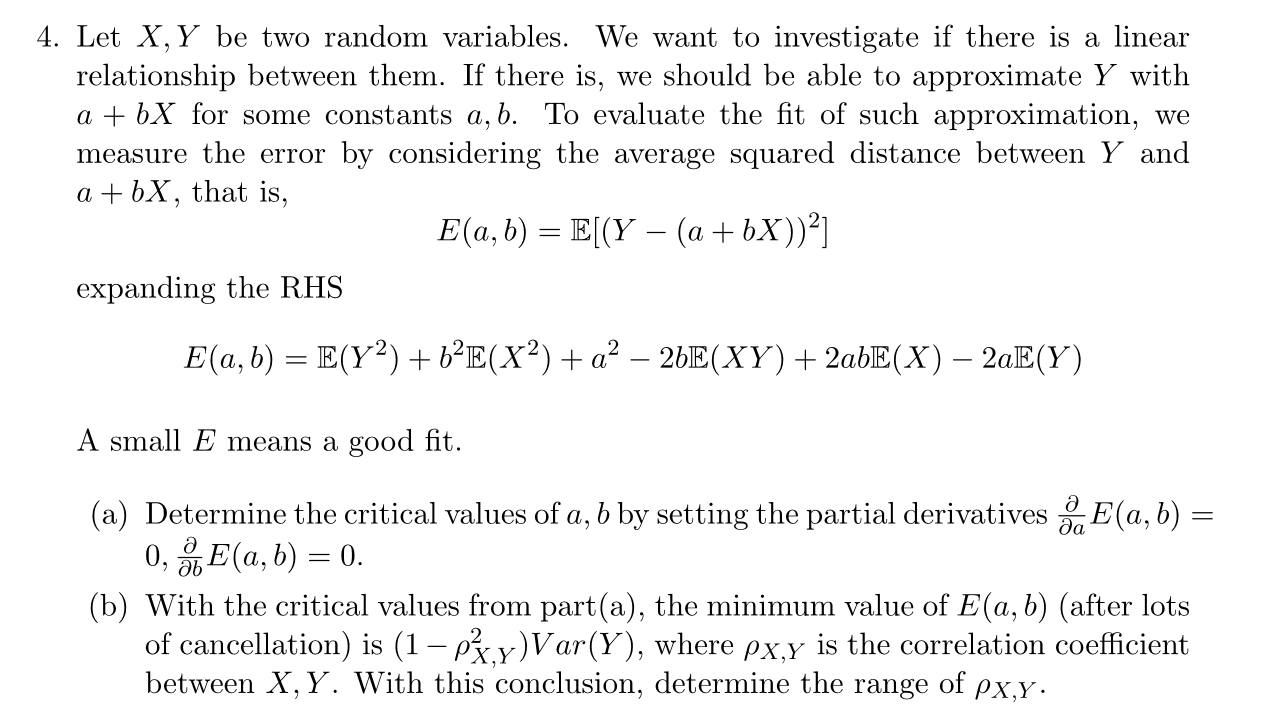# 4. Let X, Y be two random variables. We want to investigate if there is a linear relationship between them. If there is, we should be able to approximate Y witlh a + bX for some constants a,b. To evaluate the fit of such approximation, we measure the error by considering the average squared distance between Y and a + bX, that is, expanding the RHS A small E means a good fit . (a) Determine the critical values of a, b by setting the partial derivatives (a, b) (b) With the critical values from parta), the minimum value of E(a, ó) (after lots of cancellation) is (1-r2 y) Var(y), where ρxy s the correlation coefficient between X, Y. With this conclusion, determine the range of ρχ.y

Questionhelp_outlineImage Transcriptionclose4. Let X, Y be two random variables. We want to investigate if there is a linear relationship between them. If there is, we should be able to approximate Y witlh a + bX for some constants a,b. To evaluate the fit of such approximation, we measure the error by considering the average squared distance between Y and a + bX, that is, expanding the RHS A small E means a good fit . (a) Determine the critical values of a, b by setting the partial derivatives (a, b) (b) With the critical values from parta), the minimum value of E(a, ó) (after lots of cancellation) is (1-r2 y) Var(y), where ρxy s the correlation coefficient between X, Y. With this conclusion, determine the range of ρχ.y fullscreen

### Want to see this answer and more?

Experts are waiting 24/7 to provide step-by-step solutions in as fast as 30 minutes!*